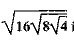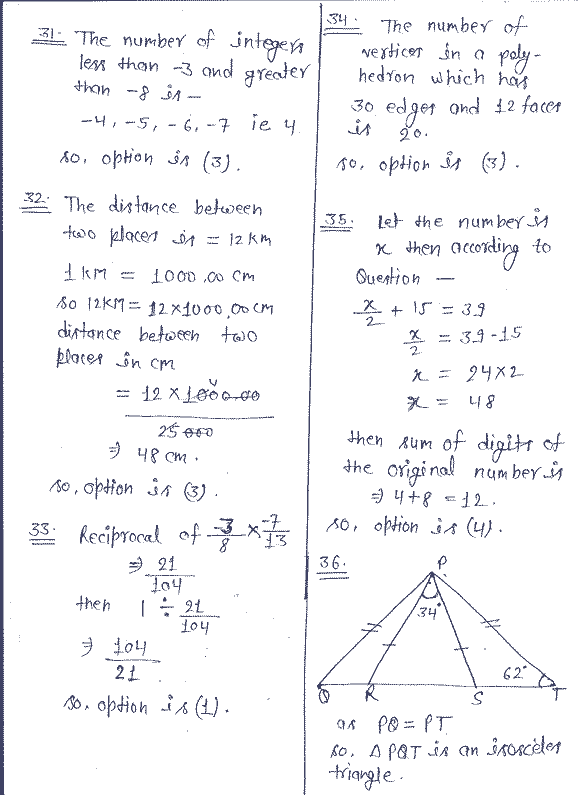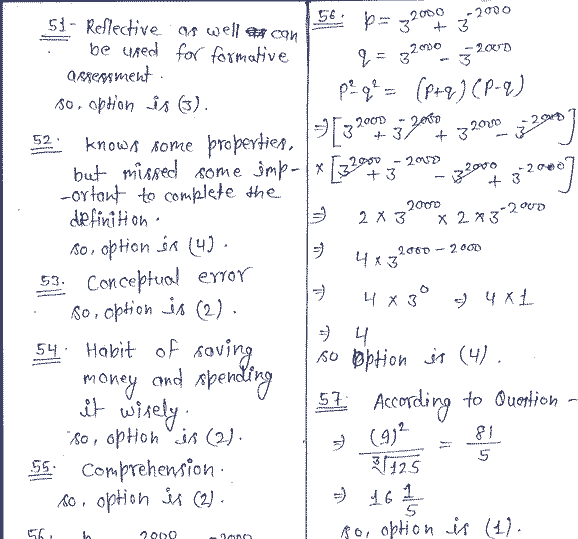Modal Question paper for CTET (Central Teacher Eligibility Test ) / TET ( Teacher Eligibility Test ) /Primary level Examination with Answer Key . There are two solved Question Paper for Math . Both are SET P (Test Booklet Code -2711205)  and SET A (Test Booklet Code -1271801)
question paper CTET July 2019 maths social science child development and pedagogy English Hindi with answer key and question paper solution provided by upjob.in official answer sheet and question paper of previous years CTET examination download PDF file

Primary Level Question Paper CTET Examination Solved question Paper

Download UPTET Answer key primary level Exam 19 December 2016 Question paper 1 solved Question paper | Solution of all set in PDF

Download UPTET Upper Primary level Question paper 2 Solved Question paper with Answer key 19 December 2016

Download Primary Upper Primary UPTET Exam Official Answer Key by Exam Regulatory Authority Up December 2016 All Set in PDF Paper 1 and paper 2 all set
MATHEMATICS MODAL PAPER FOR CTET /UPTET

CTET paper 2 question paper solution math and science upjob.in

CTET paper 2 2019 question paper solution upjob.in upper primary level

uptet 18 paper 2 math solution upjob.in

uptet 2018 question paper

31. The number of integers less than -3 but greater than -8 is
(1) 2         (2) 3        (3) 4         (4) 6

32. The distance between two places is 12 km. A map scale is 1 : 25000. The distance between the two places on the map, in cm, is
(1) 24         (2) 36        (3) 48         (4) 60

33. The reciprocal of (-3/8) * (-7/13) is;
(1) 104/21    (2) -104/21    (3) 21/104    (4) -21/104

34. The number of vertices in a polyhedron which has 30 edges and 12 faces is
(1) 12        (2) 15        (3) 20        (4) 24

35. When half of a number is increased by 15, the result is 39. The sum of digits of the original number is
(1) 6         (2).7        (3) 8        (4) 12

36. In  triangle .PQT, PQ = PT. The points R and S are on QT such that PR = PS. If angle PTS = 62° and angle RPS =34°, then measure of angle QPR is
(1) 11        (3) 15°        (2) 13°        (4) 17°

37. If for  triangle ABC and  triangle DEF, the correspondence CAB - EDF gives a congruence, then which of the followng is not true?
(1) AC=DE            (3) angle A=angle D
(2) AB=EF            (4) angle B=angle F

38. 40% of (100—20% of 300) is equal to
(1) 16        (3) 64        (2) 20        (4) 140

39. HCF of two numbers is 28 and their LCM is 336. If one number is 112, then the other number is
(1) 56        (3) 84        (2) 70        (4) 98

40. If 2/3 x = 0.6 and 0.02 y=1, then the value of x +1/y  is
(1) 0.92    (3) 49.1    (2) 1.1        (4) 50.9

41. If y= x-2/x+1 , Y does not equal to 1, then x equals
(1) y+2/1-Y    (2) y+2/Y-1    (3) Y-2/1+Y    (4) 2-Y/1-Y

42. A square and a circle have equal perimeters. The ratio of the area of the square to the area of the circle is
(1) 1:1         (2) 1:4        (3) Pai (22/7):2     (4) pai(22/7):4

43. ABCD is a square with AB = (x + 16) cm and BC = (3x) cm. The perimeter (in cm) of the square is
(1) 16         (2) 24        (3) 32         (4) 96

44. The mean of 10 numbers is 0. If 72 and -12 are included in these numbers, the new mean will be
(1) 0         (2) 5        (3) 6         (4) 60

45. The circumference of  the base of a right circular cylinder is 44 cm’ and its height is 15 cm. The volume  (in cm3) of the cylinder is ( use Pai=22/7)
(1) 770     (2) 1155    (3) 1540     (4) 2310

46. A class VII teacher wants to discuss the following problem in the class:
“A square is divided into four congruent rectangles. The perimeter of each rectangle    is 40 units. What is the perimeter of given square ?“

Key Mathematical concepts required to solve this problem is
(1) Area of square and rectangle, perimeter of square and rectangle and definition of square and rectangle
(2) Meaning of the terms square, rectangle, congruent, perimeter, etc.
(3) Area of rectangle, perimeter of square
(4) Formation of algebraic equation to solve the problem.

47. Algebra is introduced in the middle classes. According to Piagets’ theory of cognitive development, it is appropriate to introduce algebra at this stage as
(1) the child is at sensorimotor stage and can understand with the help of lots of  manipulatives.
(2) the child is at pre-operational  stage and can understand abstract concepts.
(3) the child is at concrete-operational stage and he can understand and conceptualize   concrete experiences by creating logical structure.
(4) the child is at formal operational stage and is fully mature to grasp:
the abstract concepts.

48. Mr. Sharma was assessing the students’ work on exponents. One of the response sheet was as follows:(a) 2 power 3* 2 power 5=2 power 8
(b) 3 power 2 * 4 power 2 = 12 power 4
(c) 3 power 3 ÷ 3 power 5 = 3 power -2
(d) 7 power 20 ÷7 power 14 =7 power 6
(e) 9 power 3÷18 power 6= (1/2) power 3

On the basis of this response sheet Mr. Sharma can make the following observations:
(I) Child has understood the laws of exponents and can apply them well.
(2) Child has understood the laws of exponents but has made clerical errors.
(3) Child has understood the laws of exponents but has not practiced the questions involving division of  two numbers.
(4) Child has understood the law of exponents for the cases where the base is same and has missed the   concept for the case where the base is different.

49. According to Van Hiele level of Geometric thought the five levels of geometric understanding are- visualization, analysis, informal deduction, formal deduction and rigour. Students of class VII are asked to classify the quadrilaterals according to their properties. These students are at —— level of Van Hiele Geometrical thought.
(1) Visualization
(2) Analysis
(3) Informal Deduction
(4) Formal Deduction.

50. A task assigned to the class VIII student is as follows:

An open box is to be made out of a metallic sheet of 50: cm * 65 cm. Length and breadth of the box is 30 cm and 15 cm respectively. What is the possible height of the box ? Also find the volume of this box.

(1) lower level cognitive demand as it requires the knowledge of formulae of volume of cuboid.
(2) lower level of cognitive demand as there is no connection between concepts involved and procedure required.
(3) higher level of cognitive demand as the problem can be solved by making diagrams and connections between many possible situations.
(4) higher level of cognitive demand as it requires the use of conceptual understanding that underline the procedure to complete the task.

51. Mr. Nadeem gave the following task to his class, after completing the topic on lines and angles:
Speak for 2 minutes on the following figures using your knowledge about lines and angles:(1) Reflective in nature, can be used to pass time.
(2) Exploratory in nature, can be used for summative assessments.
(3) Reflective as well can be used for formative assessment.
(4) Communicating and can encourage Mathematical Talk in the classroom during free time.

52. A child of class VII defined the rectangle as follows:

“Rectangle is a quadrilateral whose opposite sides are parallel and equal.”
The definition reflects that the child

(1) cannot recognize the shape.
(2) do not know the correct properties of the shape.
(3) knows the properties of the shape, but repeated some properties in definition.
(4) knows some properties, but missed some important to complete the definition;

53. A very common error observed in addition of linear expression is 5y+3=8y.
This type of error is termed as
(1) Clerical error
(2) Conceptual error
(3) Procedural error
(4) Careless error

54. Read the following question from the class VI text-book:

“Prabal deposited Rs 5,000 in a bank at the rate of 5% interest per - annum. After 2 years he withdrew the money to purchase the study table for Rs 3,500. He deposited the money left with him again at the rate of 5% interest per annum for another two years. How much amount will he receive after two years ?“

What values can be inculcated in students through this question?
(1) Honesty
(2) Habit of saving money spending it wisely
(3) Sincerity
(4) Simplicity and helping others

55. Following is a problem from text-book of class VI:

“Express the following statement through linear expression:
Neha has 7 more toffees than Megha. If Megha has x toffees, how many toffees does Neha have ?“
Which competence of Bloom’s cognitive domain is referred. in the above question?
(1) Knowledge     (2) Comprehension        (3) Analysis         (4) Synthesis56. If p = 3 power 2000 + 3 power -2000  and q = 3 power 2000 – 3 power -2000, then the value of p2 — q2 is(1) 1             (2) 2                (3) 3             (4) 4
57. The square of 9 is divided by the cube root of 125. The remainder is
(1) 1             (2) 2                (3) 3             (4) 4

58. The value of  square root 16 square root of 8 square root of  4 .is(1) 16              (2) 8                (3) 4               (4) 2

59. One-half of 1.2 *10 power 30 is(1) 6.0 * 10 power 30            (2) 6.0 * 10 power 29
(3) 0.6 * 5 power 30                            (4) 1.2 * 10 power 3060. If 2/3, 23/30, 9/10, 11/15 and 4/5are written in ascending order, then the fraction in
the middle most will be
(1) 23/30        (2) 4/5                 (3) 2/3             (4) 11/15

Primary level CTET Math Exam Question paper SolutionPrimary Level  CTET exam official answer key

For more news related to this, you must join our Telegram channel UPJOBIN so that you can reach the updates first.

 Ques Ans Ques Ans Ques Ans 31 3 41 1 51 3 32 3 42 4 52 4 33 1 43 4 53 2 34 3 44 2 54 2 35 4 45 4 55 2 36 1 46 2 56 4 37 2 47 3 57 1 38 1 48 4 58 2 39 3 49 2 59 2 40 1 50 4 60 1